## research papersJOURNAL OFSYNCHROTRONRADIATION
ISSN: 1600-5775
Volume 15| Part 1| January 2008| Pages 50-54

## Cost-effective upgrade of a focusing system for inelastic X-ray scattering experiments under high pressure

aNational Synchrotron Radiation Research Center, 101 Hsin-Ann Road, Hsinchu Science Park, Hsinchu 30076, Taiwan
*Correspondence e-mail: cyhuang@nsrrc.org.tw

(Received 7 September 2007; accepted 5 November 2007)

Inelastic X-ray scattering (IXS) is a powerful technique capable of probing the dynamic behavior and electronic structure of materials. For IXS experiments under high pressure up to the megabar range using state-of-the-art diamond-anvil-cell technology, the sample volume is limited to the order of 1 × 10−3 mm3 for which a beam focus of the same order and less is often required. In this paper a scheme utilizing a set of low-cost and compact Kirkpatrick–Baez mirrors for upgrading the existing optical system of the Taiwan IXS beamline at SPring-8 is described. The scheme as implemented improves the focus to 13 µm × 16 µm (horizontal × vertical) with a transmission of up to 72% and a flux density gain of over 30 times, which has enhanced substantially the efficiency of the beamline for high-pressure research.

### 1. Introduction

The properties of materials are radically altered under extreme high pressure. Chemically inert gases can be combined with other elements under high pressure (Vos et al., 1992). Carbon dioxide crystallizes into a quartz-like material (Iota et al., 1999). Moreover, most of the matter in the solar system exists under extreme high pressure. Studies of the properties of matter under high pressure will therefore extend our understanding of chemistry, material sciences, fundamental physics, geognosy and planetary sciences. For the past decades, elastic scattering techniques such as X-ray diffraction have played an important role in the structural determination of materials for high-pressure research. Inelastic X-ray scattering (IXS) techniques, on the other hand, probe directly the dynamic behavior of materials. Owing to the weak scattering cross section, IXS becomes a practical tool only with the advent of highly brilliant synchrotron sources, and is now being increasingly utilized in high-pressure research.

For experiments using state-of-the-art diamond-anvil cells (DACs) to generate static pressure up to the megabar range, the sample volume is limited to the order of 1 × 10−3 mm3. Owing to the low emittance of the brilliant synchrotron radiation and the recent development of X-ray optics, X-rays can now be focused readily to a spot comparable in size with the small sample in the DAC. IXS in conjunction with brilliant synchrotron radiation thus provides a powerful technique for studying the dynamic behavior and electronic structure of materials under megabar pressure (Mao et al., 2001). The Taiwan Contract Beamline BL12XU at SPring-8 is designed primarily for IXS experiments (Cai et al., 2004). The original optical system focuses the X-rays to about 120 µm × 80 µm [horizontal (H) × vertical (V)] at the sample position, which is sufficient for most applications under ambient conditions. For high-pressure experiments using DAC, the focusing optics of the beamline should be upgraded to produce a focal size smaller than the sample volume in the DAC. Here we describe a cost-effective scheme utilizing a set of low-cost and compact Kirkpatrick–Baez (KB) mirrors (Kirkpatrick & Baez, 1948) that focuses the X-ray further down to 13 µm × 16 µm (H × V) with a transmission of up to 72%. This translates to a flux density gain of more than 30 times. This scheme is compatible and interchangeable with the original optical system, making it possible to switch back to the original configuration for experiments that do not require the smaller focus. Furthermore, only a small transverse relocation of the focal point is introduced by the new optics, making the re-alignment of the IXS spectrometer a relatively easy task.

### 2. Optical design

The source of BL12XU is a SPring-8 standard 4.5 m-long in-vacuum undulator with a magnet period of 32 mm. The optical source size is about 720 µm × 15 µm (H × V) [full width at half-maximum (FWHM)] and the divergence is about 30 µrad × 10 µrad (H × V). The layout of the beamline optics is shown in Fig. 1. The original optical system consists of four major components including a high-heat-load Si(111) double-crystal pre-monochromator (DCM), a cylindrical collimating mirror (CM), a high-resolution monochromator (HRM) and a toroidal focusing mirror (FM). The KB mirrors placed right before the sample are the proposed microfocusing optics and will be discussed later. The DCM operates in the range from 4.5 keV to 35 keV. The CM collimates the beam vertically and rejects the higher-order light. It has two stripes: Si and Pt for the energy ranges 5–12 keV and 12–30 keV, respectively. The HRM consists of two high-precision co-axial goniometers forming various combinations of two-bounce or four-bounce (in-line or nested) channel-cut crystals. An in-line combination of two symmetric Si (333) or Si (400) channel-cut crystals is now available to provide energy resolutions of 50 meV or 153 meV, respectively, at 9.886 keV. Additional HRM crystals can be designed for different energy resolutions and scanning energy ranges. After the HRM the beam is delivered to the Pt-coated focusing mirror and focused both vertically and horizontally to 120 µm × 80 µm (H × V) at the sample position. The optical parameters of the CM and FM are listed in Table 1.

 Table 1Figure parameters of the new KB mirrors
VFM HFM
Figure Plane elliptical Plane elliptical
Coating Pt Pt
Dimensions 1 cm × 10 cm (W × L) 3 cm × 20 cm (W × L)
p, q 384.71 cm, 55.0 cm 184.67 cm, 35.0 cm
a2 1.4753 × 10−5 cm−1 3.1607 × 10−5 cm−1
a3 1.1494 × 10−7 cm−2 3.6595 × 10−7 cm−2
Slope error 1 arcsec (meridional) 1 arcsec (meridional)
Roughness 5 Å 5 Å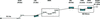Figure 1 Optical layout of BL12XU mainline.

In order to reduce the focus and at the same time retain compatibility with the origin optics, we adopted an economic approach in which the FM is detuned by changing the grazing incident angle to a moderate value. The new images of the FM are then focused to the desired size by the KB mirrors as shown in Fig. 1. For a given grazing incident angle (θ) of the toroidal FM, the object (r) and image (r′) distances are given by (Peatman, 1997)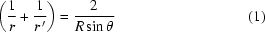for the meridional focus and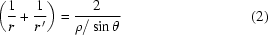for the sagittal focus, where R is the meridional curvature of the mirror and ρ is the sagittal curvature. Obviously the meridional and sagittal images are located at different positions when the FM is detuned by changing the grazing angle. As a result the required demagnifications in the horizontal and vertical directions are different. In order to focus the new images of the FM, the most sensible design is to utilize a set of KB mirrors placed before the sample (Heald, 2002). The KB arrangement which consists of a vertical focusing mirror (VFM) and a horizontal focusing mirror (HFM) can focus independently in the vertical and horizontal directions. It thus provides different demagnification in the vertical and the horizontal directions required by the highly asymmetric images of the FM.

We used Shadow (Lai & Cerrina, 1986) to simulate the focusing conditions of the FM as a function of the grazing angle. The maximum grazing angle is limited to 5 mrad in order to maintain the reflectivity to an acceptable level. The waist sizes, divergences and image distances are shown in Fig. 2. Based on the information in Fig. 2, the eventual focal size achievable by the KB mirrors can be estimated by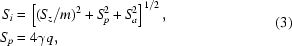where Sz is the waist size of the FM at its best focus, Sp is the contribution of the slope error, determined by the slope error γ (FWHM) and the image distance q of the KB mirrors, and Sa is the contribution of the aberration. In this case, Sa is small and can be neglected. Considering the cost, we set γ to 1 arcsec. The demagnification (m) is determined by q/p where p is the object distance of the KB mirror. Owing to the limited space of the beamline, we set the working distance of the mirror in the range 250–550 mm. Thus the object distance (p) can be calculated by |(q + r′) − 8| where r′ is the image distance of the FM and can be found in Fig. 2. The estimated focal size is shown in Fig. 3. The solid lines represent the horizontal focusing and the dotted lines represent the vertical focusing. The figure indicates that a focus of less than 20 µm × 20 µm can be achieved when the grazing angle of the FM is tuned in the range 3.0–4.3 mrad.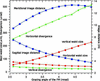Figure 2 Image distance, spot size and divergence of the FM as a function of the grazing incident angle.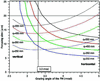Figure 3 Focal size of the KB mirrors as a function of the grazing angle of the FM at various choices of image distance.

Another key factor to consider for this design is how many photons can be collected. The mirror length determines the acceptance of the KB mirrors. The desired length of the mirror can be estimated by (Peatman, 1997)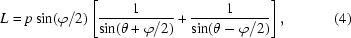where L is the length of the mirror, p is the object distance of the VFM and the HFM, and φ is the total divergence of the photon beam and is given in Fig. 2. For θ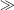φ,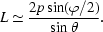Here we set φ to 6σ which is equivalent to accepting 99.7% of the photons when the reflectivity of the mirror is 100%. The calculated results are shown in Fig. 4. The solid lines are for the HFM and the dotted lines are for the VFM. For each mirror the data are branched into three groups. Group A is for the grazing angle of 5 mrad, group B is for 4 mrad and group C is for 3 mrad. Each group is composed of four lines. The blue, green, red and black lines are for q = 250 mm, q = 350 mm, q = 450 mm and q = 550 mm, respectively. q is measured from the center of the mirror to the sample position.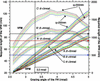Figure 4 Required mirror length of the VFM and HFM as a function of the grazing angle of the FM at various choices of the grazing angle and image distance of the KB mirrors.

To maintain a proper working distance, the mirror length must be smaller than twice the image distance (q). Owing to the small vertical divergence of the source, the desired length of the VFM is small enough to easily satisfy the condition mentioned above. For the HFM, the large horizontal divergence results in a long HFM. For example, when the grazing angle of the FM is tuned to 3.6 mrad, the desired mirror length of the HFM placed at q = 250 mm is about 760 mm for 3.0 mrad grazing incidence. It is impossible to place a 760 mm-long mirror at 250 mm in front of the sample. The length of the HFM therefore requires most of the attention in this case. Actually, when the grazing angle of the FM is tuned in the range 3.0–4.3 mrad, the only thing we need to be concerned about is the position and the length of the HFM. For convenience in choosing the combination of both, blue, green, red and black horizontal lines are depicted in the figure. These are for the lengths of 500 mm, 700 mm, 900 mm and 1100 mm, respectively. For the HFM at 250 mm from the sample, the solutions below the blue horizontal line will have an adequate length. For the HFM placed at other positions, we can find the solutions below the corresponding horizontal lines.

The geometric relation between the VFM and HFM should also be considered. In order to avoid overlapping the HFM and the VFM, the mirror length is limited by the following condition,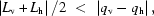where Lv is the mirror length of the VFM, Lh is the mirror length of the HFM, qv is the image distance of the VFM, and qh is the image distance of the HFM.

### 3. Ray tracing and results

Based on the above analysis, we arrive at the following configuration.

(i) The grazing angle of the FM is changed to 3.3 mrad.

(ii) The HFM of the KB mirrors is placed at 35 cm in front of the sample (center to center).

(iii) The VFM of the KB mirrors is placed at 55 cm in front of the sample.

(iv) Pt is adopted as the coating of the KB mirrors.

(v) Considering the beamline arrangement, we limit the length of the HFM to 200 mm and that of the VFM to 100 mm. Therefore the grazing angles of the VFM and HFM are set to 3.30 mrad and 3.68 mrad, respectively.

By the ray tracing via Shadow, we find the objective and image distances for the VFM and the HFM. The object and image distances of the VFM are −384.71 cm and 55.00 cm, respectively. Those of the HFM are 184.70 cm and 35.00 cm, respectively. A plane ellipse is the most suitable shape for the focusing mirror. The optimized parameters of the VFM and HFM are listed in Table 2for a plane ellipse. With the currently available technology, it is reasonable to adopt bendable KB mirrors to approach the elliptical figures (Fermé, 2002). The figure of the bendable KB mirrors can be described by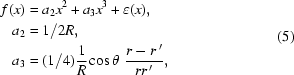where a2 is the focusing term, a3 is the elliptical term and (x) is the high-order term. R is determined by equation (1). According to the above equations, we simulated the focusing of the bendable KB mirrors via Shadow. The focusing spot at the sample position is shown in Fig. 5. Its size is about 12.6 µm × 12.6 µm (H × V). The contribution of the 1 arcsec slope errors are 10.56 µm and 6.72 µm for the VFM and the HFM, respectively. Taking into account the slope error, we obtain a focal size of 14.28 µm × 16.44 µm at the sample position. The efficiency of the KB mirrors is about 74%. The total efficiency drops to 72% because the reflectivity of the FM is degraded by the increase of the grazing angle. In comparison with the old position of the sample, the new focus declines about 3.6 mm in the vertical direction and shifts 2.6 mm in the horizontal direction. Figs. 6and 7show the results of the knife-edge scan for the focusing spot. The best focus we achieved was about 13 µm × 16 µm (H × V) which is close to our prediction, and is sufficient for the limited volume in the DAC. Recently, high-pressure experiments on SiO2 (Lin et al., 2007) and on liquid and solid He (Mao et al., 2007) have been performed successfully on the beamline.

 Table 2Optical parameters of the existing collimating and focusing mirrors
Collimating mirror Focusing mirror
Figure Cylindrical Toroidal
Coating Si/Pt Pt
Dimensions 5 cm × 70 cm (W × L) 5 cm × 70 cm (W × L)
R, ρ 4147000 cm, ∞ 640000 cm, 3.56 cm
Slope error 0.1 arcsec (meridional) 0.1 arcsec (meridional)
0.5 arcsec (sagittal) 0.5 arcsec (sagittal)
Roughness 5 Å 5 Å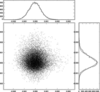Figure 5 Simulated spot size at the position of the sample.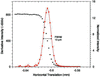Figure 6 Best achieved horizontal focus. The black dots refer to the normalized intensity and the red squares represent the derivative intensity.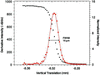Figure 7 Best achieved vertical focus. The black dots refer to the normalized intensity and the red squares represent the derivative intensity.

### ReferencesJOURNAL OFSYNCHROTRONRADIATION